##### The Japanese Bonsai specialist
Direct order Contact Help / Services Newsletter# Japanese grafting blade right handle

› Bonsai tools › Bonsai tools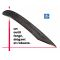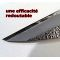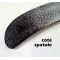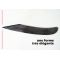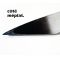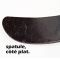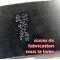ref. : 6136

45,00

Available quantity : 2Order

###### Description

Length 200 mm, width 20 mm. Measurements of the blade: 65 mm. Made of laminated steel (layers of different kinds of steel), this traditional Japanese tool is for grafting wounds. Taking the curved handle in your hand, use your thumb to push the blade upwards against the wound. A perfectly clean cut ensures proper healing of the branch.
Don't forget to view other relevant products:

http://www.maillot-bonsai.com/en/mastic_a_cicatriser_cut_pasta_pour_feuillus_160_g_-4413.php

#blade 4.5 #japanese 3.8 #grafting 3.8 #bonsai 3.6 #handle 3.6 #steel 2.5 #right 2.5 #tools 2.5 #your 2.4 #measurements 2.2

###### Technical description
 I would like to... graft my trees

Formule
(( ROUND((CHAR_LENGTH(b.article_nom)-CHAR_LENGTH(REPLACE(b.article_nom, 'blade', '')))/LENGTH('blade')) + ROUND((CHAR_LENGTH(b.article_description)-CHAR_LENGTH(REPLACE(b.article_description, 'blade', '')))/LENGTH('blade')) ) * 4.5) + (( ROUND((CHAR_LENGTH(b.article_nom)-CHAR_LENGTH(REPLACE(b.article_nom, 'japanese', '')))/LENGTH('japanese')) + ROUND((CHAR_LENGTH(b.article_description)-CHAR_LENGTH(REPLACE(b.article_description, 'japanese', '')))/LENGTH('japanese')) ) * 3.8) + (( ROUND((CHAR_LENGTH(b.article_nom)-CHAR_LENGTH(REPLACE(b.article_nom, 'grafting', '')))/LENGTH('grafting')) + ROUND((CHAR_LENGTH(b.article_description)-CHAR_LENGTH(REPLACE(b.article_description, 'grafting', '')))/LENGTH('grafting')) ) * 3.8) + (( ROUND((CHAR_LENGTH(b.article_nom)-CHAR_LENGTH(REPLACE(b.article_nom, 'handle', '')))/LENGTH('handle')) + ROUND((CHAR_LENGTH(b.article_description)-CHAR_LENGTH(REPLACE(b.article_description, 'handle', '')))/LENGTH('handle')) ) * 3.6) + (( ROUND((CHAR_LENGTH(b.article_nom)-CHAR_LENGTH(REPLACE(b.article_nom, 'right', '')))/LENGTH('right')) + ROUND((CHAR_LENGTH(b.article_description)-CHAR_LENGTH(REPLACE(b.article_description, 'right', '')))/LENGTH('right')) ) * 2.5) + (( ROUND((CHAR_LENGTH(b.article_nom)-CHAR_LENGTH(REPLACE(b.article_nom, 'steel', '')))/LENGTH('steel')) + ROUND((CHAR_LENGTH(b.article_description)-CHAR_LENGTH(REPLACE(b.article_description, 'steel', '')))/LENGTH('steel')) ) * 2.5) + (( ROUND((CHAR_LENGTH(b.article_nom)-CHAR_LENGTH(REPLACE(b.article_nom, 'your', '')))/LENGTH('your')) + ROUND((CHAR_LENGTH(b.article_description)-CHAR_LENGTH(REPLACE(b.article_description, 'your', '')))/LENGTH('your')) ) * 2.4) + (( ROUND((CHAR_LENGTH(b.article_nom)-CHAR_LENGTH(REPLACE(b.article_nom, 'measurements', '')))/LENGTH('measurements')) + ROUND((CHAR_LENGTH(b.article_description)-CHAR_LENGTH(REPLACE(b.article_description, 'measurements', '')))/LENGTH('measurements')) ) * 2.2) + (( ROUND((CHAR_LENGTH(b.article_nom)-CHAR_LENGTH(REPLACE(b.article_nom, 'traditional', '')))/LENGTH('traditional')) + ROUND((CHAR_LENGTH(b.article_description)-CHAR_LENGTH(REPLACE(b.article_description, 'traditional', '')))/LENGTH('traditional')) ) * 2.1) + (( ROUND((CHAR_LENGTH(b.article_nom)-CHAR_LENGTH(REPLACE(b.article_nom, 'cicatriser', '')))/LENGTH('cicatriser')) + ROUND((CHAR_LENGTH(b.article_description)-CHAR_LENGTH(REPLACE(b.article_description, 'cicatriser', '')))/LENGTH('cicatriser')) ) * 2)

## Secure payment## Delivery

Our logistic partners :04 74 55 23 48
Pépinière MAILLOT-BONSAÏ
Le Bois Frazy
01990 RELEVANT - FRANCE
on appointment